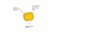# Why electric field is zero inside a conductor?

• ddnath

#### ddnath

1.Why electric field is zero inside a conductor? Can anyone explain it with Gauss's law and without Gauss's law?

2.Is it possible to create electric field inside a conductor? If so,how and in which condition it is created?

1. In electrostatics the electric field is 0 inside a perfect conductor because otherwise there would be moving charges. The charges must redistribute themselves to make a net electric field inside the conductor 0.

2. It is possible to have an electric field inside a conductor. You can put one there by e.g. using a battery. The trick is that this prompts charges to move inside the conductor and therefore a current to develop. This is no longer electrostatics.

Well,I got this.But how you will explain this with Gauss's law?Explain why electric field is zero inside a conductor by the help of Gauss's law.

Now if i ask,why charges are always distributed on the surface?Why there is no charge inside the conductor?I prepared like this...

Consider a gaussian surface inside the conductor A (see the figure attached).As electric field is zero inside the conductor,Gauss's law requires that there could be no net charge inside the surface.Now imagine shrinking the surface like a collapsing balloon until it encloses a region so small that we may consider it as a point.Then the charge at that point must be zero.We can do this anywhere inside the conductor,so there could be no excess charge an any point within a solid conductor.Any excess charge must reside on the conductor surface.

#### Attachments

•gauss law in conductor.jpg
5.3 KB · Views: 2,145
Gauss' Law can not be used since as stated that is for an electrostatic condition. In other words it is not used when you have a moving charge. If you have a charge within an electric field that puts a force on that charge. If it is free to move it will move due to that force. In your illustration each charge would create its own electric field that other charges will react to. All of the charges push against all of the other charges and they end up on the outer boundary of the conductor where they cannot move away from each other any further.

Ohm's law (differential form) $\textbf{j}=\sigma\textbf{E}$
If E is not zero, then a current will very quickly form in a conductor. If the conductor is surrounded by insulator, then charge can't escape from the conductor, so you can't have net current escaping from the conductor. Therefore, the flux of E over the surface of the conductor is 0.

There's no law that says E is zero inside the conductor, but it goes to 0 very quickly in steady state or slowly varying conditions. Internal E field will generate internal currents, which will quickly dissipate electrical energy into heat via internal resistance.

•Sho Kano
Lines of force cancel each other

We all know that the charge enclosed by a surface gives the sum of electirc lines of force.BUt we have to remember that lines of force can cancel each other.Keeping that in mind and also the fact that,an isolated conductor has chaged particles oriented in random direction,the lines of force can cancel each other and hence integrating the lines force over a surface integral,we get 0